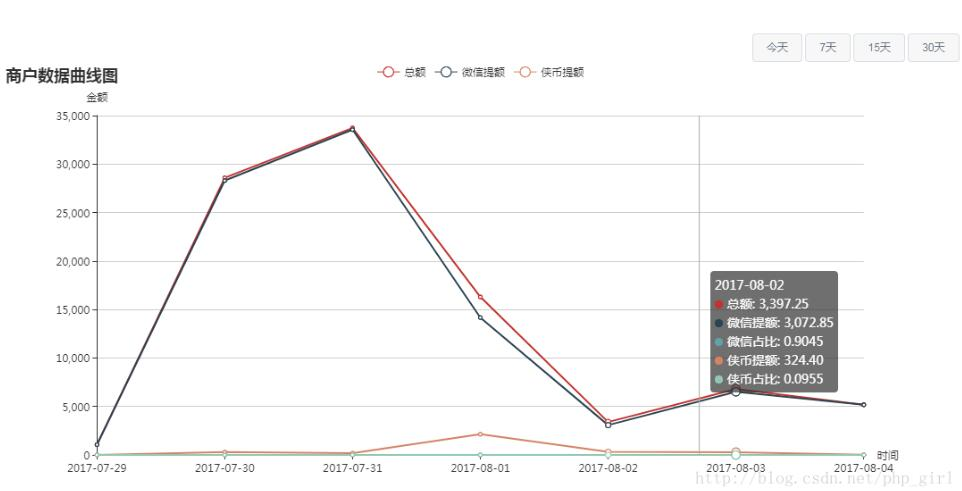laravel实现按月或天或小时统计mysql数据的方法

2019-10-09 18:07 44 查看1. 控制器内容

/**
* [getsellerdata 获取某时间段内商户结算查询数据]
* @param Request \$request [description] start:起始时间 end:结束时间
* @return [type]      [description]
*/
public function getsellerqudata(Request \$request){
\$data = \$this->dataanalysis->getSellerQuData(\$request->start,\$request->end);
return \$data;
}

2. 库文件内容

/**
* [getSellerQuData 获取商户结算数据 曲线]
* @param [string] \$start [起始时间]2017-08
* @param [string] \$end  [结束时间]
* @return [type]    [description]
*/
public function getSellerQuData(\$name,\$start,\$end){

//计算时间差值,以决定格式化时间格式
\$diff = strtotime(\$end)-strtotime(\$start);

//分组条件 1天内按小时分组,否则按天/月分组
//86400/1天 2678400/1月
if(\$diff<86400&&\$diff>0){
\$sort = '%H';
}elseif(\$diff<2678400){
\$sort = '%Y-%m-%d';
}else{
\$sort = '%Y-%m';
}
//把数据添加时间按格式化时间分组求和,求和分两种,一种是直接求和,一种是满足case when条件的数据求和
\$query = DB::table('user_withdrawals as w')->select(DB::raw("FROM_UNIXTIME(created_at,'{\$sort}') as thedata,sum(case when w.cash_type = 1 then w.money end) as xiabi,sum(case when w.cash_type = 2 then w.money end) as online,sum(w.money) as alls"))->groupBy(DB::raw("FROM_UNIXTIME(created_at,'{\$sort}')"));

//条件筛选 某时间段内
if( !empty(\$start) ){
\$query->whereRaw('w.created_at >= ?',strtotime(\$start));
}
if( !empty(\$end) ){
\$query->whereRaw('w.created_at <= ?',strtotime(\$end));
}

\$data = \$query->get();

return \$data;
}